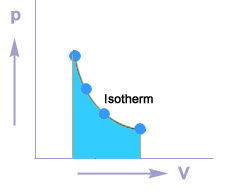# Isothermal Process

Isothermal process
A thermodynamic process in which the temperature of the system remains constant during the supply of heat is called an isothermal process.

Isothermal compression
Consider a cylinder of non-conducting walls and good heat conducting base. The cylinder is fitted with a frictionless piston. An ideal gas is enclosed in the cylinder. In the first stage pressure on the piston is increased and the cylinder is placed on a cold body. Due to compression , the temperature of the system increases but at the same time DQ amount of heat is removed from the system and the temperature of the system is maintained.
According to the first law of thermodynamics:
DQ = DU+ DW
Since temperature is constant, therefore, there is no change in internal energy of the system. i.e. DU = 0
As the work is done on the system, therefore, DW is negative,
DQ = 0+ (-DW)
DQ = –DW

Isothermal expansion
in another situation the cylinder is placed over a hot body and the pressure on the system is decreased. Due to expansion, the temperature of the system is decreased but at the same time DQ amount of heat is absorbed from the hot body and the temperature of the system is again maintained.
According to the first law of thermodynamics:
DQ = DU+ DW
Since temperature is constant, therefore, there is no change in internal energy of the system. i.e. DU = 0
As the work is done by the system, therefore, DW is positive,
DQ = 0+ (DW)
DQ = +DW